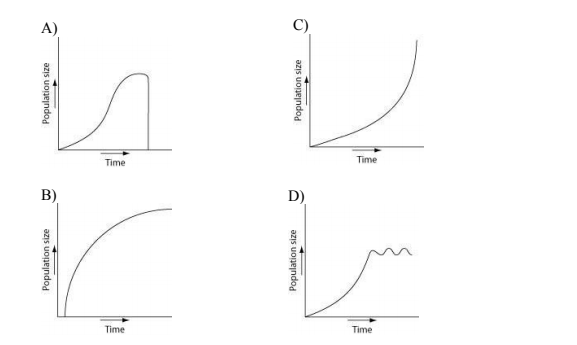# Problem: Which of the following graphs illustrates the population growth curve starting with a single bacterium growing in a flask of ideal medium at optimum temperature over a two-hour period? Assume resources do not become limiting over this time frame.

###### Problem Details

Which of the following graphs illustrates the population growth curve starting with a single bacterium growing in a flask of ideal medium at optimum temperature over a two-hour period? Assume resources do not become limiting over this time frame.What scientific concept do you need to know in order to solve this problem?

Our tutors have indicated that to solve this problem you will need to apply the Population Ecology concept. You can view video lessons to learn Population Ecology. Or if you need more Population Ecology practice, you can also practice Population Ecology practice problems.

What is the difficulty of this problem?

Our tutors rated the difficulty ofWhich of the following graphs illustrates the population gro...as high difficulty.

How long does this problem take to solve?

Our expert Biology tutor, Kaitlyn took 5 minutes and 38 seconds to solve this problem. You can follow their steps in the video explanation above.

What professor is this problem relevant for?

Based on our data, we think this problem is relevant for Professor Calhoon's class at OSU.